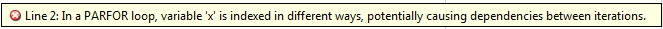Documentation

## Ensure That `parfor`-Loop Iterations are Independent

If you get an error when you convert `for`-loops to `parfor`-loops, ensure that your `parfor`-loop iterations are independent. `parfor`-loop iterations have no guaranteed order, while the iteration order in `for`-loops is sequential. Also `parfor`-loop iterations are performed on different MATLAB® workers in the parallel pool, so that there is no sharing of information between iterations. Therefore one `parfor`-loop iteration must not depend on the result of a previous iteration. The only exception to this rule is to accumulate values in a loop using Reduction Variables.

The following example produces equivalent results, using a `for`-loop on the left and a `parfor`-loop on the right. Try the example in your MATLAB Command Window:

 ```clear A for i = 1:8 A(i) = i; end A``` ```A = 1 2 3 4 5 6 7 8 ``` ```clear A parfor i = 1:8 A(i) = i; end A``` ```A = 1 2 3 4 5 6 7 8 ```

Each element of `A` is equal to its index. The `parfor`-loop works because each element is determined by the indexed loop variable only and does not depend on other variables. `for`-loops with independent tasks are ideal candidates for `parfor`-loops.

### Note

By default, `parfor` automatically starts a parallel pool of workers, if you have not started one already. `parfor` creates a pool using your default cluster profile, if you have set your parallel preferences accordingly.

In the example, the array elements are available in the client workspace after the `parfor`-loop, exactly as with a `for`-loop.

Now use a nonindexed variable inside the loop, or a variable whose indexing does not depend on the loop variable `i`. Try these examples, and note the values of `d` and `i` afterward:

 ```clear A d = 0; i = 0; for i = 1:4 d = i*2; A(i) = d; end A d i``` ```A = 2 4 6 8 d = 8 i = 4``` ```clear A d = 0; i = 0; parfor i = 1:4 d = i*2; A(i) = d; end A d i``` ```A = 2 4 6 8 d = 0 i = 0```

Although the elements of `A` are the same in both examples, the value of `d` is not. In the `for`-loop, the iterations are executed sequentially, so afterward `d` has the value it held in the last iteration of the loop. In the `parfor`-loop, however, the iterations execute in parallel, so it is impossible to assign `d` a defined value at the end of the loop. This situation also applies to the loop variable `i`. Therefore, `parfor`-loop behavior is defined so that it does not affect the values `d` and `i` outside the loop. Their values remain the same before and after the loop. If the variables in your `parfor`-loop are not independent, then you might get different answers from those in the `for`-loop. In summary, a `parfor`-loop requires that each iteration be independent of the other iterations. All code that follows the `parfor` statement should not depend on the loop iteration sequence.

Code Analyzer can help diagnose whether the loop iterations are dependent. The code in the example shows iterations defined in terms of the previous iteration:

``` parfor k = 2:10 x(k) = x(k-1) + k; end ```
Look for Code Analyzer messages in the MATLAB Editor. In this case, Code Analyzer reports the dependency problem.In other cases, however, Code Analyzer is unable to mark dependencies.

For help with other common `parfor` problems, see Nested parfor and for-Loops and Other parfor Requirements.